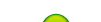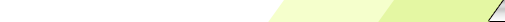Friday, 2020-02-28BioInfo Pakistan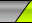Home | Sign Up | Log In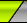Welcome Guest | RSS
Section categories
 Related Subjects This category includes brief overview of all related subjects. Defining BioInformatics In this section we tried to briefly explain what bioinformatics is ? Unviersities This contains information about universities that are offering bioinformatics degree programs. Resources Contains information about bioinformatics resources including databases, tools and techniques. Algorithms This category includes some of the basic algorithms that are usually used by bioinformaticians.
 Our poll Pakistani Student Yes No Total of answers: 0
 Chat Box Only authorized users can post messages
 StatisticsTotal online: 1 Guests: 1 Users: 0
Home » 2011 » August » 15 » Kabsch Algorithm
9:31 PM
Kabsch Algorithm

 Kabsch Algorithm* From Wikipedia, the free encyclopedia1. Introduction:   The Kabsch algorithm, named after Wolfgang Kabsch, is a method for calculating the optimal rotation matrix that minimizes the RMSD (root mean squared deviation) between two paired sets of points. It is useful in graphics, cheminformatics to compare molecular structures, and also bioinformatics for comparing protein structures.The algorithm only computes the rotation matrix, but it also requires the computation of a translation vector. When both the translation and rotation are actually performed, the algorithm is sometimes called partial Procrustes superimposition.2. Description:   The algorithm starts with two sets of paired points, P and Q. Each set of points can be represented as an N×3 matrix. The first row is the coordinates of the first point, the second row is the coordinates of the second point, the Nth row is the coordinates of the Nth point.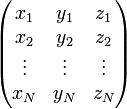The algorithm works in three steps: a translation, the computation of a covariance matrix, and the computation of the optimal rotation matrix.TranslationBoth sets of coordinates must be translated first, so that their centroid coincides with the origin of the coordinate system. This is done by subtracting from the point coordinates the coordinates of the respective centroid.Computation of the covariance matrixThe second step consist of calculating a covariance matrix A. In matrix notation,A = PTQor, using summation notation,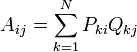Computation of the optimal rotation matrixIt is possible to calculate the optimal rotation U based on the matrix formula U = (ATA)1 / 2A − 1 but implementing a numerical solution to this formula becomes complicated when all special cases are accounted for (for example, the case of A not having an inverse).If singular value decomposition (SVD) routines are available, the optimal rotation, U, can be calculated using the following simple algorithm.First, calculate the SVD of the covariance matrix A.A = VSWTNext, decide whether we need to correct our rotation matrix to insure a right-handed coordinate systemd = sign(det(A))Finally, calculate our optimal rotation matrix, U, as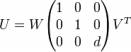GeneralizationsThe algorithm was described for points in a three dimensional space. The generalization to D dimensions is immediate.
Category: Algorithms | Views: 1887 | Added by: Ansari | Rating: 0.0/0